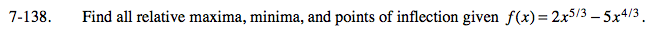### Home > CALC > Chapter 7 > Lesson 7.3.3 > Problem7-138

7-138.Candidates for relative maxima and minima occur where f'(x) = 0 and f '(x) = DNE.
Candidates for points of inflection occur where f "(x) = 0 and f "(x) = DNE.
But these are just CANDIDATES.
You have to conduct other tests before indentifying each candidate as the location of a max, min or POI.

Once you determine the x-value of each relative max, relative min and POI, recall that maxima and minima are defined as y-values.
Use the function f(x) to determine the corresponding y-coordinates.